Open In App
Related Articles
• Write an Interview Experience
• Deep Learning Tutorial

# Introduction to Recurrent Neural Network

In this article, we will introduce a new variation of neural network which is the Recurrent Neural Network also known as (RNN) that works better than a simple neural network when data is sequential like Time-Series data and text data.

## What is Recurrent Neural Network (RNN)?

Recurrent Neural Network(RNN) is a type of Neural Network where the output from the previous step is fed as input to the current step. In traditional neural networks, all the inputs and outputs are independent of each other, but in cases when it is required to predict the next word of a sentence, the previous words are required and hence there is a need to remember the previous words. Thus RNN came into existence, which solved this issue with the help of a Hidden Layer. The main and most important feature of RNN is its Hidden state, which remembers some information about a sequence. The state is also referred to as Memory State since it remembers the previous input to the network. It uses the same parameters for each input as it performs the same task on all the inputs or hidden layers to produce the output. This reduces the complexity of parameters, unlike other neural networks.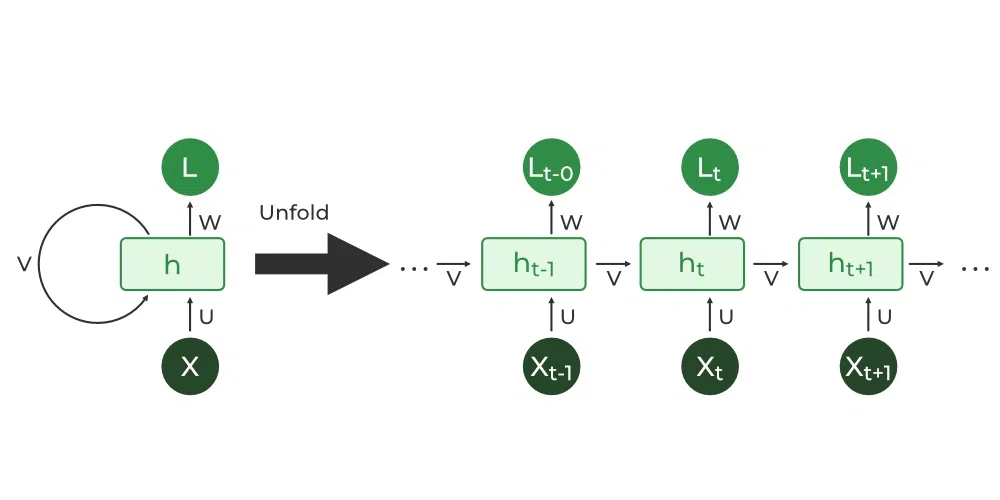REcurrent neural network

## Architecture Of Recurrent Neural Network

RNNs have the same input and output architecture as any other deep neural architecture. However, differences arise in the way information flows from input to output. Unlike Deep neural networks where we have different weight matrices for each Dense network in RNN, the weight across the network remains the same. It calculates state hidden state  Hi for every input Xi . By using the following formulas:

h= σ(UX + Wh-1 + B)

Y = O(Vh + C) Hence

Y = f (X, h , W, U, V, B, C)

Here S is the State matrix which has element si as the state of the network at timestep i
The parameters in the network are W, U, V, c, b which are shared across timestep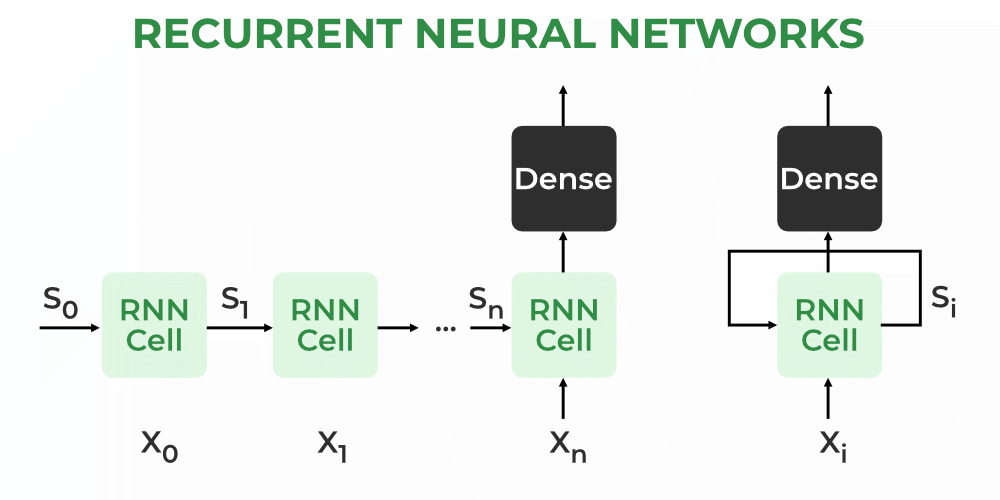What is Recurrent Neural Network

### How  RNN works

The Recurrent Neural Network consists of multiple fixed activation function units, one for each time step. Each unit has an internal state which is called the hidden state of the unit. This hidden state signifies the past knowledge that the network currently holds at a given time step. This hidden state is updated at every time step to signify the change in the knowledge of the network about the past. The hidden state is updated using the following recurrence relation:-

The formula for calculating the current state: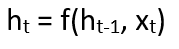where:

ht -> current state
ht-1 -> previous state
xt -> input state

Formula for applying Activation function(tanh):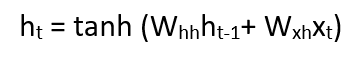where:

whh -> weight at recurrent neuron
wxh -> weight at input neuron

The formula for calculating output: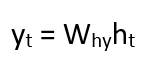Yt -> output
Why -> weight at output layer

These parameters are updated using Backpropagation. However, since RNN works on sequential data here we use an updated backpropagation which is known as Backpropagation through time.

### Backpropagation Through Time (BPTT)

In RNN the neural network is in an ordered fashion and since in the ordered network each variable is computed one at a time in a specified order like first h1 then h2 then h3 so on. Hence we will apply backpropagation throughout all these hidden time states sequentially.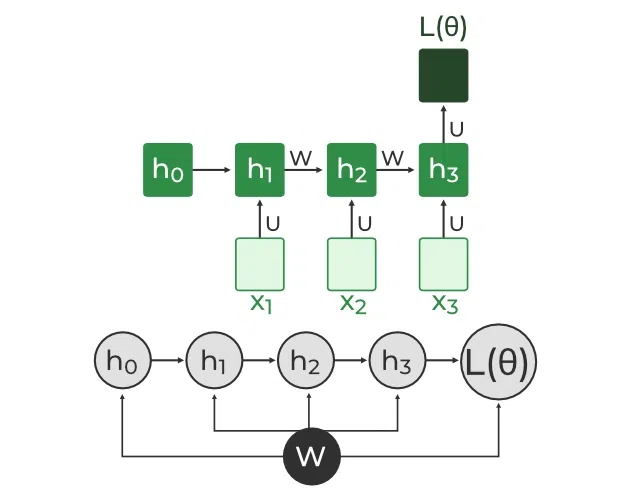Backpropagation Through Time (BPTT) In RNN

L(θ)(loss function) depends on h3
h3 in turn depends on h2 and W
h2 in turn depends on h1 and W
h1 in turn depends on h0 and W
where h0 is a constant starting state.For simplicity of this equation, we will apply backpropagation on only one rowWe already know how to compute this one as it is the same as any simple deep neural network backpropagation.However, we will see how to apply backpropagation to this termAs we know h3 = σ(Wh2 + b)

And In such an ordered network, we can’t computeby simply treating h3 as a constant because as it also depends on W. the total derivativehas two parts

1. Explicit:treating all other inputs as constant
2. Implicit: Summing over all indirect paths from h3 to W

Let us see how to do thisFor simplicity, we will short-circuit some of the pathsFinally, we haveWhereHence,This algorithm is called backpropagation through time (BPTT) as we backpropagate over all previous time steps

### Training through RNN

1. A single-time step of the input is provided to the network.
2. Then calculate its current state using a set of current input and the previous state.
3. The current ht becomes ht-1 for the next time step.
4. One can go as many time steps according to the problem and join the information from all the previous states.
5. Once all the time steps are completed the final current state is used to calculate the output.
6. The output is then compared to the actual output i.e the target output and the error is generated.
7. The error is then back-propagated to the network to update the weights and hence the network (RNN) is trained using Backpropagation through time.

### Advantages of Recurrent Neural Network

1. An RNN remembers each and every piece of information through time. It is useful in time series prediction only because of the feature to remember previous inputs as well. This is called Long Short Term Memory.
2. Recurrent neural networks are even used with convolutional layers to extend the effective pixel neighborhood.

1. Gradient vanishing and exploding problems.
2. Training an RNN is a very difficult task.
3. It cannot process very long sequences if using tanh or relu as an activation function.

Applications of Recurrent Neural Network

1. Language Modelling and Generating Text
2. Speech Recognition
3. Machine Translation
4. Image Recognition, Face detection
5. Time series Forecasting

## Types Of RNN

There are four types of RNNs based on the number of inputs and outputs in the network.

1. One to One
2. One to Many
3. Many to One
4. Many to Many

### One to One

This type of RNN behaves the same as any simple Neural network it is also known as Vanilla Neural Network. In this Neural network, there is only one input and one output.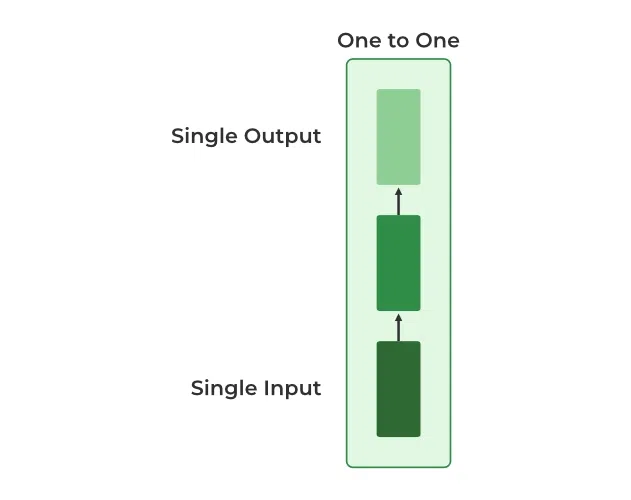One to One RNN

### One To Many

In this type of RNN, there is one input and many outputs associated with it. One of the most used examples of this network is Image captioning where given an image we predict a sentence having Multiple words.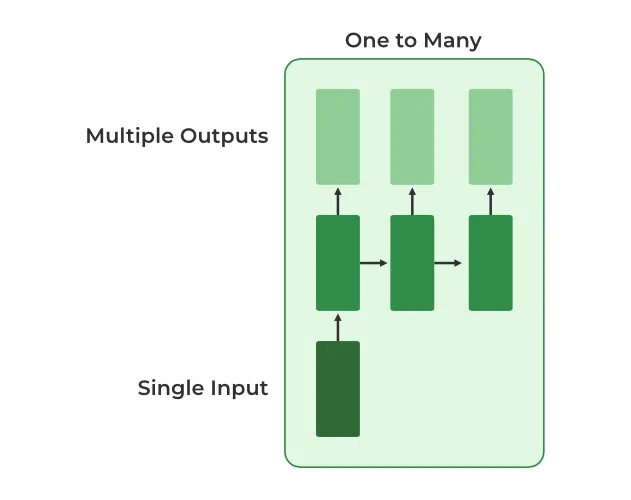One To Many RNN

### Many to One

In this type of network, Many inputs are fed to the network at several states of the network generating only one output. This type of network is used in the problems like sentimental analysis. Where we give multiple words as input and predict only the sentiment of the sentence as output.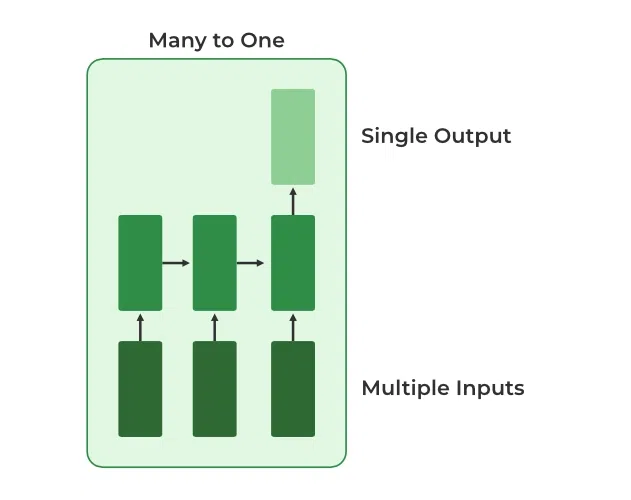Many to One RNN

### Many to Many

In this type of neural network, there are multiple inputs and multiple outputs corresponding to a problem. One Example of this Problem will be language translation. In language translation, we provide multiple words from one language as input and predict multiple words from the second language as output.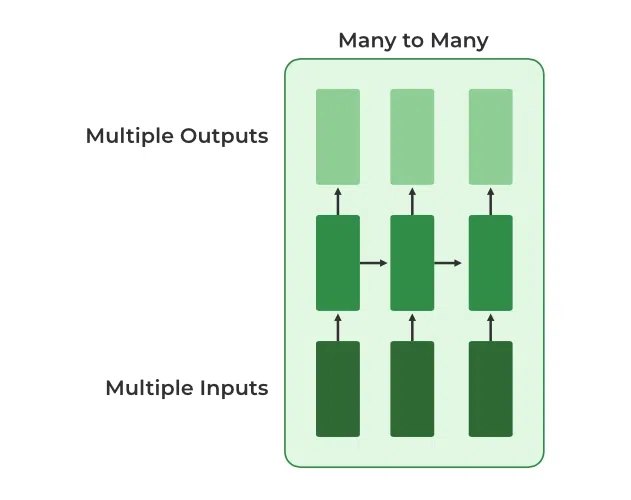Many to Many RNN

## Variation Of Recurrent Neural Network (RNN)

To overcome the problems like vanishing gradient and exploding gradient descent several new advanced versions of RNNs are formed some of these are as ;

1. Bidirectional Neural Network (BiNN)
2. Long Short-Term Memory (LSTM)

Bidirectional Neural Network (BiNN)

A BiNN is a variation of a Recurrent Neural Network in which the input information flows in both direction and then the output of both direction are combined to produce the input. BiNN is useful in situations when the context of the input is more important such as Nlp tasks and Time-series analysis problems.

Long Short-Term Memory (LSTM)

Long Short-Term Memory works on the read-write-and-forget principle where given the input information network reads and writes the most useful information from the data and it forgets about the information which is not important in predicting the output. For doing this three new gates are introduced in the RNN. In this way, only the selected information is passed through the network.

## Difference between RNN and Simple Neural Network

RNN is considered to be the better version of deep neural when the data is sequential. There are significant differences between the RNN and deep neural networks  they are listed as: